## A buoy is a floating device that can have many purposes, but often as a locator for ships. Collin constructs a hollow metal buoy by welding

Question

A buoy is a floating device that can have many purposes, but often as a locator for ships. Collin constructs a hollow metal buoy by welding together two identical cones of height h and diameter d. The resulting double-cone buoy has average density equal to half the density of seawater meaning that its total mass divided by total volume is half the density of seawater. Throughout this problem, you can ignore the air above the sea’s surface.1. When the buoy is at rest in a calm ocean, with its axis of symmetry perpendicular to the water’s surface, what fraction of it will be submerged?2. If the buoy is at rest at time t = 0 and is then pushed down slightly into the water and let go, what will be its angular frequency ω of small oscillations in terms of the given variables? Note: the volume of a cone of height h and base area A is hA/3.3. What should be the height h of each cone in the buoy so that the buoy will execute one oscillation period every second and therefore be usable as a clock with one-second accuracy?

in progress 0
6 months 2021-07-28T03:21:38+00:00 1 Answers 6 views 0

A) since the density of the buoy is half the density of sea water when the buoy is at rest on an ocean half of the buoy will be submerged in water

B )angular frequency ( w ) =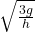c )  h = 1.34 m ( 4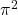/ 3g )

Explanation:

A) since the density of the buoy is half the density of sea water when the buoy is at rest on an ocean half of the buoy will be submerged in water this is because the substances with lesser density floats when placed in a substance with a higher density

B ) when the Buoy is at rest ( t = 0 ) and is then pushed down calculate angular frequency ( w ) of small oscillations in terms of the given variables

volume of cone = hA /3.

h = height of cone, A = Base Area.

therefore the total volume of the Buoy above water level ( at rest )

= (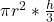) * 2 =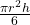The  part of the buoy immersed in water = x

then the net upward force will be

fb ( force of Buoy ) =  fg ( force of gravity )

note force = Mass * Acceleration

force of buoy =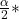$$\frac{\pi r^{2} }{h}$$* a = ( force of gravity )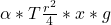therefore a =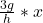angular frequency ( w ) =C ) height of the each cone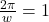therefore w = 2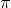back to the angular frequency :therefore h = 1.34 m ( 4/ 3g )